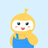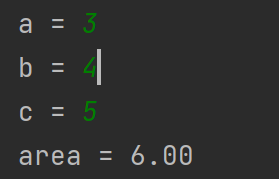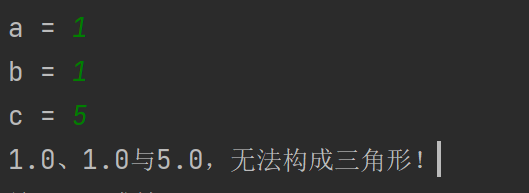• 如何用C语言编程序：输入三个数，判断是否构成三角形
千次阅读
2021-05-19 12:24:27

满意答案wl3080342

2013.04.23采纳率：48%    等级：12

已帮助：5482人

这个是我新编的,你可以试试

#include

#include

void main()

{

float a,b,c,d,s;

scanf("%f,%f,%f",&a,&b,&c);

if(a+b>c&&a+c>b&&b+c>a)

{

if(a==b||b==c||c==a)

{if(a==b&&b==c) printf("this is a dengbiansanjiaoxing\n"); else printf("this is a dengyao sanjiaoxing\n"); }

else if(a*a+b*b==c*c||a*a+c*c==b*b||b*b+c*c==a*a)

printf("this is zhijiao\n");

else printf("yibansanjiaoxing\n");

d=(a+b+c)/2;

s=sqrt(d*(d-a)*(d-b)*(d-c));

printf("%f\n",s);

}

}

else printf("bushi sanjiaoxing ") ;

}

12分享举报

更多相关内容
• 给定平面上任意三个点的坐标(x1,y1)、(x2,y2)、(x3,y3)，检验它们能否构成三角形。 解体思路：构成三角形的要求是，两边之和大于第三条边，读者根据这个准则判定即可。 C语言源代码演示： #include<...

给定平面上任意三个点的坐标(x1,y1)、(x2,y2)、(x3,y3)，检验它们能否构成三角形。

解体思路：构成三角形的要求是，两边之和大于第三条边，读者根据这个准则判定即可。

C语言源代码演示：

#include <stdio.h>//头文件
#include <math.h>//为了引用sqrt函数
int main()//主函数
{
double x1,y1,x2,y2,x3,y3;//定义变量
float side_Length1,side_Length2,side_Length3;//定义变量

printf("请输入第一个坐标；");//提示语句
scanf("%lf %lf",&x1,&y1);
printf("请输入第二个坐标；");//提示语句
scanf("%lf %lf",&x2,&y2);
printf("请输入第三个坐标；");//提示语句
scanf("%lf %lf",&x3,&y3);

side_Length1=sqrt(pow(x2-x1,2)+pow(y2-y1,2));
side_Length2=sqrt(pow(x3-x1,2)+pow(y3-y1,2));
side_Length3=sqrt(pow(x3-x2,2)+pow(y3-y2,2));

if(side_Length1+side_Length2>side_Length3&&side_Length2+side_Length3>side_Length1
&&side_Length1+side_Length3>side_Length2) //判断条件
{
printf("这三个点可以构成三角形！\n");
}
else
{
printf("这三个点无法构成三角形！\n");
}
return 0;//主函数返回值为0
}

编译运行结果：

请输入第一个坐标；1 0
请输入第二个坐标；4 0
请输入第三个坐标；2 2
这三个点可以构成三角形！

--------------------------------
Process exited after 7.599 seconds with return value 0
请按任意键继续. . .

今天的分享就到这里了，大家要好好学C语言/C++哟~

写在最后：对于准备学习C/C++编程的小伙伴，如果你想更好的提升你的编程核心能力（内功）不妨从现在开始！

C语言C++编程学习交流圈子，QQ群：763855696【点击进入】

C语言从入门到精通（C语言入门C语言教程C语言零基础C语言基础C语言学习C

整理分享（多年学习的源码、项目实战视频、项目笔记，基础入门教程）

欢迎转行和学习编程的伙伴，利用更多的资料学习成长比自己琢磨更快哦！

编程学习视频分享：展开全文c语言 c++
• 三角形面积 from math import sqr a = float(input('a = ')) b = float(input('b = ')) c = float(input('c = ')) if a + b > c and b + c > a and c + a > b: p = (a + b + c) / 2 area = sqrt(p * (p...

求三角形面积

from math import sqr
a = float(input('a = '))
b = float(input('b = '))
c = float(input('c = '))
if a + b > c and b + c > a and c + a > b:
p = (a + b + c) / 2
area = sqrt(p * (p - a) * (p - b) * (p - c))
print('area = {:.2f}'.format(area))
else:
print('{}、{}与{}，无法构成三角形！'.format(a, b, c))展开全文html 前端 css
• Apple iPhone 11 (A2223) 128GB 黑色 移动联通电信4G手机 双卡双待4999元包邮去购买 >输入三角形的三边，判断是否构成三角形。若能构成输出yes，否则输出no。输入格式:在一行中直接输入3个整数，3个整数之间各用一...

Apple iPhone 11 (A2223) 128GB 黑色 移动联通电信4G手机 双卡双待

4999元包邮

去购买 >输入三角形的三边，判断是否能构成三角形。若能构成输出yes，否则输出no。

输入格式:

在一行中直接输入3个整数，3个整数之间各用一个空格间隔，没有其他任何附加字符。

输出格式:

直接输出yes或no，没有其他任何附加字符。

代码如下：

#!/usr/bin/python

# -*- coding: utf-8 -*-

s = list(input().split())

x,y,z = int(s),int(s),int(s)

if z > x and z > y and x+y > z and z-y < x and z-x < y:

print("yes")

elif x > z and x >y and z + y > x and x-y < z and x-z < y:

print("yes")

elif y > z and y >y and x+z > y and y-z < x and y-x < z:

print("yes")

else :

print("no")

这个程序实现起来不难，不过我写的有点烦乱，后期在网上看看有没有简便的吧。

读书和健身总有一个在路上展开全文• 入的三个边长(正整型值)，fun函数判断能否构成三角形，若能构成三角形则返回1,若不能构成三角形则返回0。判断方法是任意两边之和大于第三边则构成三角形，否则不能。例如，输入"12,4,9"，则输出"可以构成三角形"。代码
• 主要介绍了Python判断三段线能否构成三角形的代码，具有很好的参考价值，希望对大家有所帮助。一起跟随小编过来看看吧
• #include int orsanjiaoxing(float n1, float n2, float n3); int orsanjiaoxing(float n1, float n2, float n3) { int a; a=(n1+n2) > n3 && (n1+n3)>n2 ...printf("可以构成三角形") :printf("不可以构成三角形"); }c语言
• 三角形面积 ‪‬‪‬‪‬‪‬‪‬‮‬‭‬‫‬‪‬‪‬‪‬‪‬‪‬‮‬‪‬‫‬‪‬‪‬‪‬‪‬‪‬‮‬‪‬‮...输入三角形的三条边a、b、c，判断三条边是否能够构成三角形，如果能够构成三角形，则输出该三角形..python
• 这个判断是否构成三角形的代码有bug,请问bug是什么,怎么修改? ` is_triangle = function(a,b,c){ triple = c(a,b,c) max_index = which.max(triple) cond = (triple[max_index](triple[-max_index])) ...r语言 r语言
• 输入三角形的三边，判断是否构成三角形。若能构成输出yes，否则输出no。 输入格式: 在一行中直接输入3个整数，3个整数之间各用一个空格间隔，没有其他任何附加字符。 输出格式: 直接输出yes或no，没有其他任何...
• 【C语言初学】输入三条边判断是否构成三角形以及三角形的种类c语言 开发语言 后端
• 本来是比较容易的题目，但是一旦要考虑到非法输入，会比较复杂。 程序 #include <iostream> #include <vector> #include <string> #include <algorithm> #include <... string nuc++
• # 三角形判断三角形，等边、等腰、直角 # def triangle(x, y, z): equilateral_triangle = f'{x, y, z}构成等边三角形' isosceles_triangle = f'{x, y, z}构成等腰三角形' right_triangle = f'{x, y, z}构成...python
• #include<stdio.h> #include<math.h> float area(int a,int b,int c) { float s,l; l=(a+b+c)/2.0;...s=sqrt(l*(l-a)(l-b)(l-c));//海伦公式 计算三角形的面积 ...a)//判断是否构成三角形 tc语言
• 输入三角形的三边，判断是否构成三角形。若能构成输出yes，否则输出no。 输入格式: 在一行中直接输入3个整数，3个整数之间各用一个空格间隔，没有其他任何附加字符。 输出格式: 直接输出yes或no，没有其他任何附加...python
• 7-3 jmu-python-判断是否构成三角形 (10 分) 输入三角形的三边，判断是否构成三角形。若能构成输出yes，否则输出no。 输入格式: 在一行中直接输入3个整数，3个整数之间各用一个空格间隔，没有其他任何附加字符。...python
• def triangle(a,b,c): ... print('输入的三条边符合组成三角形的编程规则！') a=float(input('请输入边长 a:')) b=float(input('请输入边长 b:')) c=float(input('请输入边长 c:')) triangle(a,b,c)python
• 由题意得：要判断三条边得长度是否组成一个三角形 条件为：任意两边的和要大于第三边，任意两边的差要小于第三边 注意：求差时要取绝对值 代码如下 import java.util.Scanner; import static java.lang.Math.abs;...java 算法 数学
• Description：输入三角形的三边，判断是否构成三角形。若能构成输出yes，否则输出no。 Input：输入仅一行，输入输入3个整数，3个整数之间各用一个空格间隔，没有其他任何附加字符。 Output：输出仅一行，输出直接...python 算法
• Python : 7-10 jmu-python-判断是否构成三角形 （10 分） 输入三角形的三边，判断是否构成三角形。若能构成输出yes，否则输出no。 输入格式: 在一行中直接输入3个整数，3个整数之间各用一个空格间隔，没有...Python
• #include<stdio.h> main() { float a,b,c; printf("请输入三个边边长\n"); scanf("%f%f%f",&a,&b,&c); if(a+b>c&&...printf("等边三角形\n");...printf("等腰三角形\n")
• 从键盘输入三角形的三条边长，判断是否构成三角形，如能则求出三角形的周长和面积并输出；如不能，输出不能构成三角形的信息。构成三角形的条件为：三角形任意两边的和大于第三边时，构成三角形。 算法分析： #...
• 判断能否构成三角形 题目描述 输入三角形的三条边a、b、c的值，判断能否构成三角形。 输入 输入3个整数，表示三条边长，判断能否构成三角形。 输出 如果能构成三角形，则输出Yes；不能，则输出No。 样例输入 【输入...c++...